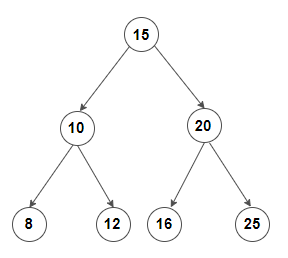# Find Inorder Predecessor for given key in a BST

Given a BST, find in-order predecessor of a given key in it. If the given key do not lie in the BST, then return the previous greater key (if any) present in the BST.

An in-order predecessor of a node in BST is the previous node in in-order traversal of it. For example, consider below treein-order predecessor of 8 do not exist.
in-order predecessor of 10 is 8
in-order predecessor of 12 is 10
in-order predecessor of 20 is 16

A node’s in-order predecessor is node with maximum value in its left sub tree i.e. its left subtree’s right-most child. If left subtree of the node doesn’t exists, then in-order predecessor is one of the ancestors of it. To find which ancestors is the predecessor, we can move up the tree towards root until we encounter a node which is right child of it’s parent. If any such node is found, the in-order predecessor is its parent else in-order predecessor do not exists for the node.

We can recursively check above conditions. The idea is to search for given node in the tree and update the predecessor to current node before visiting its right subtree. If the node is found in the BST, then we return maximum value node in its left subtree and if the left subtree of the node doesn’t exists, then in-order predecessor is one of the ancestors of it which is already been updated while searching for the given key.

Below is C++/Java implementation of the idea:

## Java

Output:

Predecessor of node 15 is 12
Predecessor of node 10 is 8
Predecessor of node 20 is 16
Predecessor doesn’t exists for node 8
Predecessor of node 12 is 10
Predecessor of node 16 is 15
Predecessor of node 25 is 20

The time complexity of above solution is O(n).

#### Iterative version –

The same algorithm can be easily implemented iteratively.

Below is C++/Java implementation of the idea:

## Java

Output:

Predecessor of node 15 is 12
Predecessor of node 10 is 8
Predecessor of node 20 is 16
Predecessor doesn’t exists for node 8
Predecessor of node 12 is 10
Predecessor of node 16 is 15
Predecessor of node 25 is 20

The time complexity of above solution is O(n) and auxiliary space used by the program is O(1).(2 votes, average: 5.00 out of 5)Loading...

Please use our online compiler to post code in comments. To contribute, get in touch with us.
Like us? Please spread the word and help us grow. Happy coding 🙂Subscribe
Notify ofGuest

Awesome!!Guest

I believe your algorithm is too over complicated, you don’t need to handle the case when the current element is equal to the target one:Guest
alexkidd

Hi there, both solutions produce output instead of NULL when the key does not exist as value 🙂